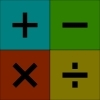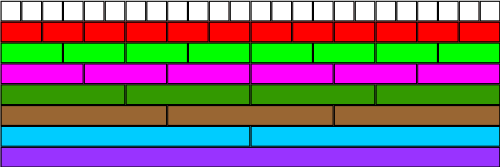#### You may also like### Worms

Place this "worm" on the 100 square and find the total of the four squares it covers. Keeping its head in the same place, what other totals can you make?### Which Scripts?

There are six numbers written in five different scripts. Can you sort out which is which?### Highest and Lowest

Put operations signs between the numbers 3 4 5 6 to make the highest possible number and lowest possible number.

##### Age 7 to 11Challenge Level

You may like to try our Fractional Wall problem before this one.Using the fraction wall above, can you say which is bigger, $\frac{1}{3}$ or $\frac{2}{8}$? By how much?

Which is smaller, $\frac{5}{6}$ or $\frac{3}{4}$? By how much?

What is the difference between $\frac{5}{6}$ and $\frac{1}{3}$?

What is three quarters of $\frac{2}{3}$? Can you explain how you worked this out?